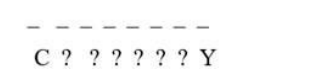# How many words beginning with C and ending with Y can be formed by

Question:

How many words beginning with C and ending with Y can be formed by using the letters of the word ‘COURTESY’?

Solution:

To find: number of words starting with C and end with Y

There are 8 letters in word COURTESY.

Here the position of the letters $C$ and $Y$ are fixed which is $1^{\text {st }}$ and $8^{\text {th }}$.Rest 6 letters are to be arranged in 6 places which can be done in $P(6,6)$.

Formula:

Number of permutations of $n$ distinct objects among $r$ different places, where repetition is not allowed, is

$P(n, r)=n ! /(n-r) !$

Therefore, a permutation of 6 different objects in 6 places is

$P(6,6)=\frac{6 !}{(6-6) !}=\frac{6 !}{0 !}=\frac{720}{1}=720$

Therefore, total number of words starting with C and ending with Y is 720.